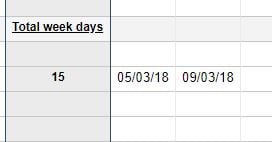# Calculating Days

Hello

How do i get this formula to Calculating the numbers of Days when i select the date columns.

e.g I want to select the dates and then it calculates the number of days from the two date ranges selected.